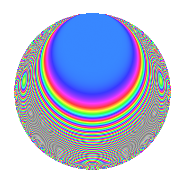# Properties

 Label 288.2.sLevel 288 Weight 2 Character orbit s Rep. character $$\chi_{288}(95,\cdot)$$ Character field $$\Q(\zeta_{6})$$ Dimension 24 Newform subspaces 1 Sturm bound 96 Trace bound 0

# Related objects

## Defining parameters

 Level: $$N$$ = $$288 = 2^{5} \cdot 3^{2}$$ Weight: $$k$$ = $$2$$ Character orbit: $$[\chi]$$ = 288.s (of order $$6$$ and degree $$2$$) Character conductor: $$\operatorname{cond}(\chi)$$ = $$36$$ Character field: $$\Q(\zeta_{6})$$ Newform subspaces: $$1$$ Sturm bound: $$96$$ Trace bound: $$0$$

## Dimensions

The following table gives the dimensions of various subspaces of $$M_{2}(288, [\chi])$$.

Total New Old
Modular forms 112 24 88
Cusp forms 80 24 56
Eisenstein series 32 0 32

## Trace form

 $$24q - 4q^{9} + O(q^{10})$$ $$24q - 4q^{9} + 8q^{21} + 12q^{25} + 24q^{29} - 20q^{33} - 36q^{41} - 8q^{45} + 12q^{49} - 36q^{57} - 48q^{65} - 16q^{69} + 24q^{73} - 48q^{77} - 20q^{81} - 64q^{93} + 12q^{97} + O(q^{100})$$

## Decomposition of $$S_{2}^{\mathrm{new}}(288, [\chi])$$ into newform subspaces

Label Dim. $$A$$ Field CM Traces $q$-expansion
$$a_2$$ $$a_3$$ $$a_5$$ $$a_7$$
288.2.s.a $$24$$ $$2.300$$ None $$0$$ $$0$$ $$0$$ $$0$$

## Decomposition of $$S_{2}^{\mathrm{old}}(288, [\chi])$$ into lower level spaces

$$S_{2}^{\mathrm{old}}(288, [\chi]) \cong$$ $$S_{2}^{\mathrm{new}}(36, [\chi])$$$$^{\oplus 4}$$$$\oplus$$$$S_{2}^{\mathrm{new}}(144, [\chi])$$$$^{\oplus 2}$$

## Hecke Characteristic Polynomials

There are no characteristic polynomials of Hecke operators in the database